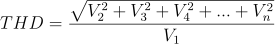# Total Harmonic Distortion

The Total Harmonic Distortion (THD) is defined as the ratio between the power of the harmonic frequencies above the base frequency and the power of the base frequency. This ratio is displayed in dB. It is a measure of the distortion in a signal.

The THD is calculated using the following formula:where V1 is the RMS amplitude of the base frequency and V2 to Vn are the RMS amplitudes of each higher harmonic.

The THD measurement can only be used on frequency based signals or spectra.